Courses

# Introduction: The Slope Deflection Method - 2 Civil Engineering (CE) Notes | EduRev

## Civil Engineering (CE) : Introduction: The Slope Deflection Method - 2 Civil Engineering (CE) Notes | EduRev

The document Introduction: The Slope Deflection Method - 2 Civil Engineering (CE) Notes | EduRev is a part of the Civil Engineering (CE) Course Structural Analysis.
All you need of Civil Engineering (CE) at this link: Civil Engineering (CE)

Application of Slope-Deflection Equations to Statically Indeterminate Beams.

The procedure is the same whether it is applied to beams or frames. It may be summarized as follows:

1. Identify all kinematic degrees of freedom for the given problem. This can be done by drawing the deflection shape of the structure. All degrees of freedom are treated as unknowns in slope-deflection method.
2. Determine the fixed end moments at each end of the span to applied load. The table given at the end of this lesson may be used for this purpose.
3. Express all internal end moments in terms of fixed end moments and near end, and far end joint rotations by slope-deflection equations.
4. Write down one equilibrium equation for each unknown joint rotation. For example, at a support in a continuous beam, the sum of all moments corresponding to an unknown joint rotation at that support must be zero. Write down as many equilibrium equations as there are unknown joint rotations.
5. Solve the above set of equilibrium equations for joint rotations.
6. Now substituting these joint rotations in the slope-deflection equations evaluate the end moments.
7. Determine all rotations.

Example 14.1
A continuous ABC beam is carrying uniformly distributed load of 2 kN/m in addition to a concentrated load of 20 kN as shown in Fig.14.5a. Draw bending moment and shear force diagrams. Assume EI to be constant.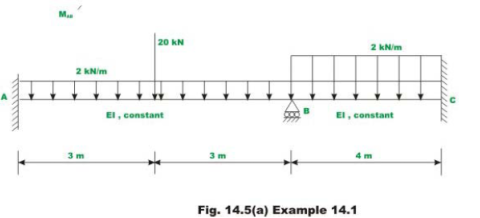(a). Degrees of freedom
It is observed that the continuous beam is kinematically indeterminate to first degree as only one joint rotation θB is unknown. The deflected shape /elastic curve of the beam is drawn in Fig.14.5b in order to identify degrees of freedom. By fixing the support or restraining the support B against rotation, the fixed-fixed beams area obtained as shown in Fig.14.5c.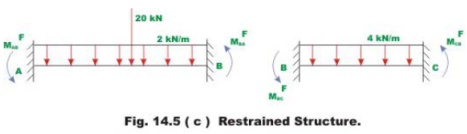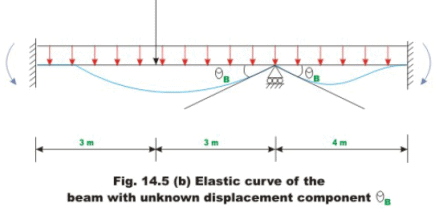(b). Fixed end moments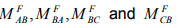are calculated referring to the Fig. 14. and following the sign conventions that counterclockwise moments are positive.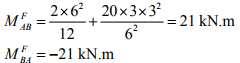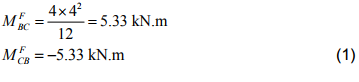(c) Slope-deflection equations
Since ends A and C are fixed, the rotation at the fixed supports is zero, θA = θC = 0. Only one non-zero rotation is to be evaluated for this problem. Now, write slope-deflection equations for span AB and BC.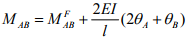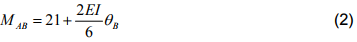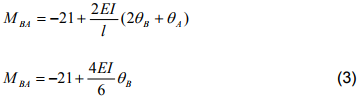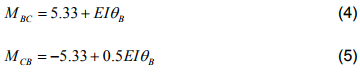(d) Equilibrium equations
In the above four equations (2-5), the member end moments are expressed in terms of unknown rotation θB . Now, the required equation to solve for the rotation θB is the moment equilibrium equation at support B. The free body diagram of support B along with the support moments acting on it is shown in Fig. 14.5d. For, moment equilibrium at supportB, one must have,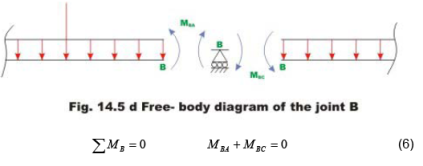Substituting the values of MBA and MBC in the above equilibrium equation,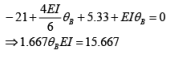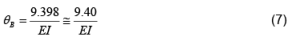(e) End moments
After evaluating θB , substitute it in equations (2-5) to evaluate beam end moments. Thus,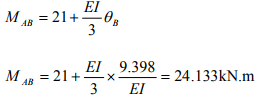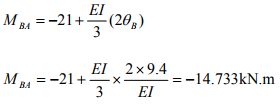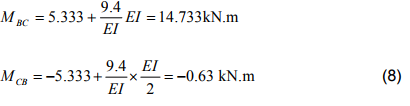(f) Reactions
Now, reactions at supports are evaluated using equilibrium equations (vide Fig. 14.5e)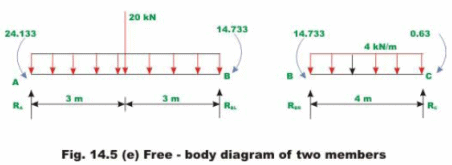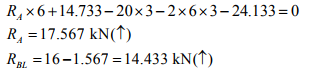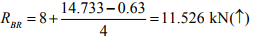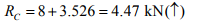(9)

The shear force and bending moment diagrams are shown in Fig. 14.5f.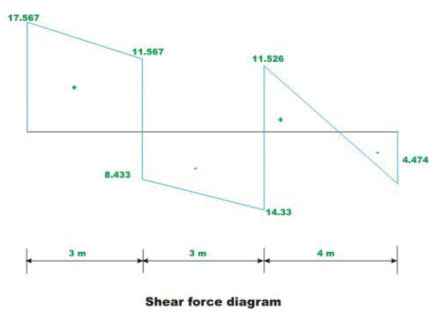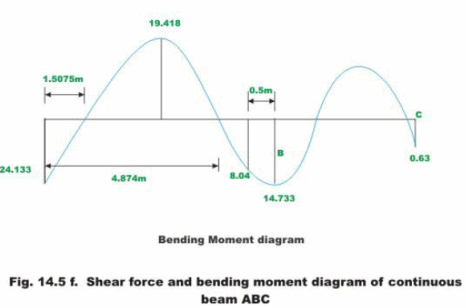Example 14.2

Draw shear force and bending moment diagram for the continuous beam loaded as shown in Fig.14.6a.The relative stiffness of each span of the beam is also shown in the figure.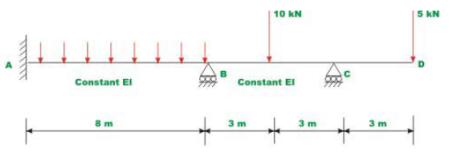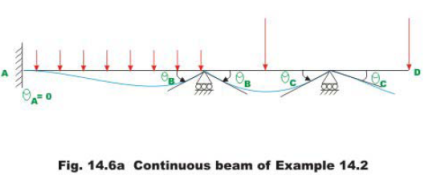For the cantilever beam portion CD, no slope-deflection equation need to be written as there is no internal moment at end D. First, fixing the supports at B and C, calculate the fixed end moments for span AB and BC. Thus,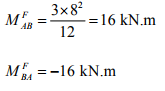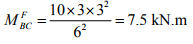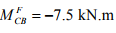(1)

In the next step write slope-deflection equation. There are two equations for each span of the continuous beam.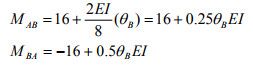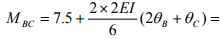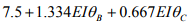MBC = -7.5 +1334EIθC + 0.667EIθB                               (2)

Equilibrium equations The free body diagram of members AB , BC and joints B and C are shown in Fig.14.6b.One could write one equilibrium equation for each joint B and C.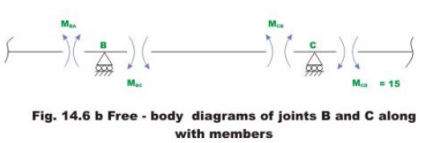Support B,

∑MB = 0      MBA + MBC = 0                           (3)

∑MC = 0      MCB + MCD = 0                            (4)

We know MCD that 15 kN.m                            (5)

⇒ MCB =− 15 kN.m                                           (6)

Substituting the values of MCB and MCD in the above equations for MAB ,MBA ,MBC and MCB we get,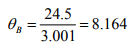θC = 9.704                                 (7)

Substituting θBC in the slope-deflection equations, we get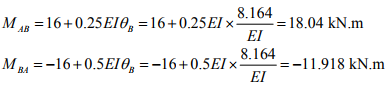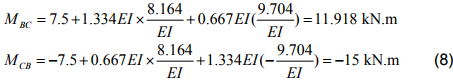Reactions are obtained from equilibrium equations (ref. Fig. 14.6c)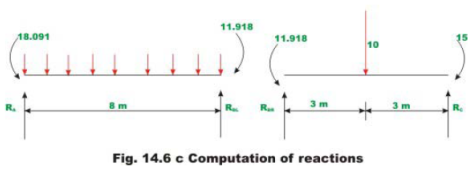RA x 8 -18.041 - 3 x 8 x 4 +11.918 = 0

Ra = 12.765 kN

RBR = 5 - 0.514kN = 4.486 kN

RBL = 11.235 kN

RC = 5 + 0.514kN = 5.514 kN

The shear force and bending moment diagrams are shown in Fig. 14.6d.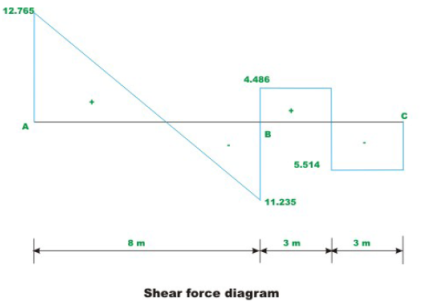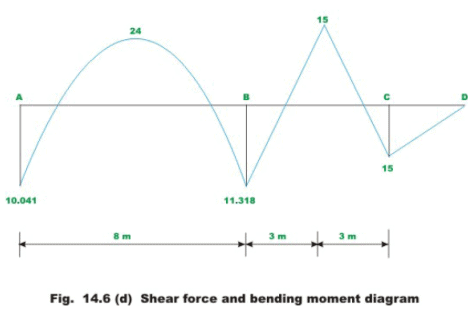For ease of calculations, fixed end forces for various load cases are given in Fig. 14.7.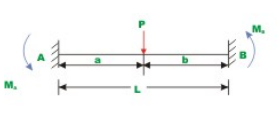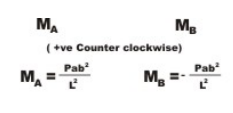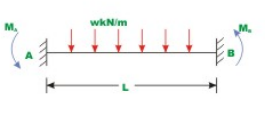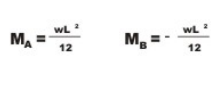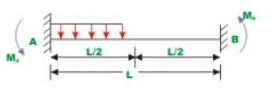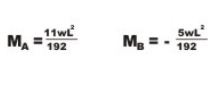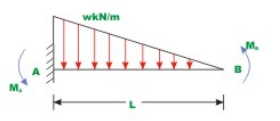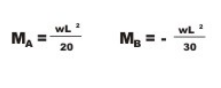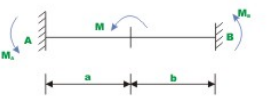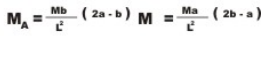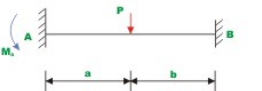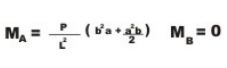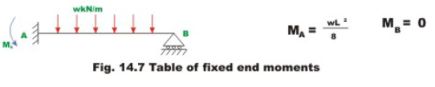Summary

In this lesson the slope-deflection equations are derived for beams with unyielding supports. The kinematically indeterminate beams are analysed by slope-deflection equations. The advantages of displacement method of analysis over force method of analysis are clearly brought out here. A couple of examples are solved to illustrate the slope-deflection equations.

Offer running on EduRev: Apply code STAYHOME200 to get INR 200 off on our premium plan EduRev Infinity!

## Structural Analysis

30 videos|122 docs|28 tests

,

,

,

,

,

,

,

,

,

,

,

,

,

,

,

,

,

,

,

,

,

;